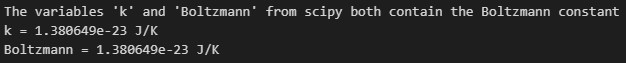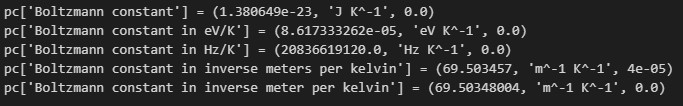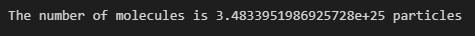## Python Boltzmann Constant

##### Aniekan

One of the most fundamental physical constants in science is the Boltzmann constant. It connects the temperature, energy and thermodynamic properties of a gas. How can we make use of Boltzmann’s constant in Python? This article will show us how to access it, and give a sample calculation using Python Boltzmann constant. So let’s get started.

## What’s the Boltzmann constant?

The Boltzmann constant (denoted by k or k_b) is a fundamental physical constant in Physics and Chemistry. It plays an important role in connecting the temperature, energy, and thermodynamic properties of gases. The constant is named after the Austrian Physicist Ludwig Boltzmann, who made significant contributions to statistical mechanics.

The Boltzmann constant relates the average kinetic energy of gas particles to the temperature of the gas. It has dimensions of energy divided by temperature; its unit of measure is in Joules per Kelvin (J/K), with a numerical value of 1.380649×10^{-23} J/K. This means that for each Kelvin increase in temperature, the increase in energy is going to be of the minute amount 1.380649×10^{-23} Joules. Even though this quantity seems tiny, it can become significant when considering large-scale systems.

The Boltzmann constant appears in various equations and formulae such as Boltzmann’s entropy formula, Plank’s law of black-body radiation, and the ideal gas law. It is also useful in calculating thermal noise in resistors.

However, if we need to carry out scientific computations that require the use of this constant, how can we access the Boltzmann constant in Python

## How to access Python Boltzmann constant

The Boltzmann constant is not an inbuilt constant in Python. So, in order to make use of it, we can simply define a variable and assign to it the value of the constant. However, the scipy package defines this constant for our convenience. So, in order to make use of the constant, we have to install scipy into our virtual environment. We can do this by running the following command on our console:

pip install scipy

Then, we import the scipy.constants module. This module provides us with a wide variety of physical constants. Here, however, we are interested in the Boltzmann constant. the code snippet below shows us how to access the constant:

from scipy.constants import k, Boltzmann

print(f"The variables 'k' and 'Boltzmann' from scipy both contain the Boltzmann constant")
print(f"{k = } J/K")
print(f"{Boltzmann = } J/K")

The code snippet above demonstrates how to import and use the Boltzmann constant from the scipy.constants module. The scipy.constants uses both the k and Boltzmann variables to represent the Boltzmann constant, and they can be used interchangeably. The output is shown below:As seen in the code output, they contain the same value; the Boltzmann constant in J/K units.

## The constant in other units

The defined variables in scipy (k and Boltzmann) have the Boltzmann constant in the unit J/K. In other to make use of the Boltzmann constant in other units, we can avoid the stress of converting it ourselves since scipy provides the constant in other units for our convenience. But, how do we access them?

We can import the dictionary physical_constants from scipy. This dictionary contains a comprehensive collection of physical constants. The keys are descriptive of the names of the constant names, reflecting the names they are recognized by in the scientific community.

The code snippet below shows how to access the Boltzmann constant defined in other units (scipy version 1.10.0):

from scipy.constants import physical_constants as pc

print(f"{pc['Boltzmann constant'] = }")         # default in units J/K
print(f"{pc['Boltzmann constant in eV/K'] = }")
print(f"{pc['Boltzmann constant in Hz/K'] = }")
print(f"{pc['Boltzmann constant in inverse meters per kelvin'] = }")
print(f"{pc['Boltzmann constant in inverse meter per kelvin'] = }")

The physical_constants dictionary is named with the convenient alias pc. The value returned for each access is a tuple having the form (value of constant, unit of measure, uncertainty). The calls to the print function display each of the defined variations of the Boltzmann constant that come with scipy 1.10.0. The actual printout is shown below:As the output shows, each constant is displayed along with its value and unit.

## Example calculation using Python Boltzmann constant

Now comes one practical application of the Boltzmann constant; the ideal gas law. The ideal gas law describes the relationship between the pressure (P), volume (V), temperature (T), and number of moles (n) of an ideal gas. Mathematically:

PV=nRT

Where R is the constant of proportionality called the universal gas constant, or molar gas constant, and its value equals 8.31446261815324 J\cdot K^{−1}\cdot mol{−1}.

If we want to find the number of molecules in a gas (rather than the number of moles) under the same conditions of pressure, temperature, and volume, we can make use of The Boltzmann constant, and modify the ideal gas equation as follows:

PV=NkT

In this case of the equation, P, V, and T, take their previous meanings. However, there are a few changes:

• We now have N (number of molecules) rather than n (number of moles).
• The constant used has changed from R to Boltzmann’s constant k.

An example problem is as follows:

Find the number of molecules in 2.00 L of gas at 35.0 \degree C and under 7.41 \times 10^7 N/m^2 of pressure.

We can carry out the calculation in Python as follows:

from scipy.constants import k

# Pressure: Is already in Pascals
P = 7.41e7

# Volume: Convert from Litres to cubic metres
V = 2.00 / 1000

# Temperature: Convert from Celsius to Kelvin
T = 35.0 + 273.15

# Calculate the value of N
N = (P*V)/(k*T)

print(f"The number of molecules is {N} particles")

The code first carries out some conversions to make sure the given quantities are in compatible units. Finally, the number of molecules is calculated using the formula

N=\dfrac{PV}{kT}

and the resulting value is printed out with the following output:In conclusion, we have considered the Boltzmann constant, an important physical constant that connects temperature, energy, and the thermodynamic properties of gases. We have also seen how to use it in Python by using the defined constant in the scipy package.

scipy.constants module also makes it convenient to make use of the constant without the need for conversions.

Finally, we carried out an example calculation involving the ideal gas law.

If you liked this tutorial, please feel free to check out our Python Tutor to assist you in learning how to solve coding problems.

Happy coding!